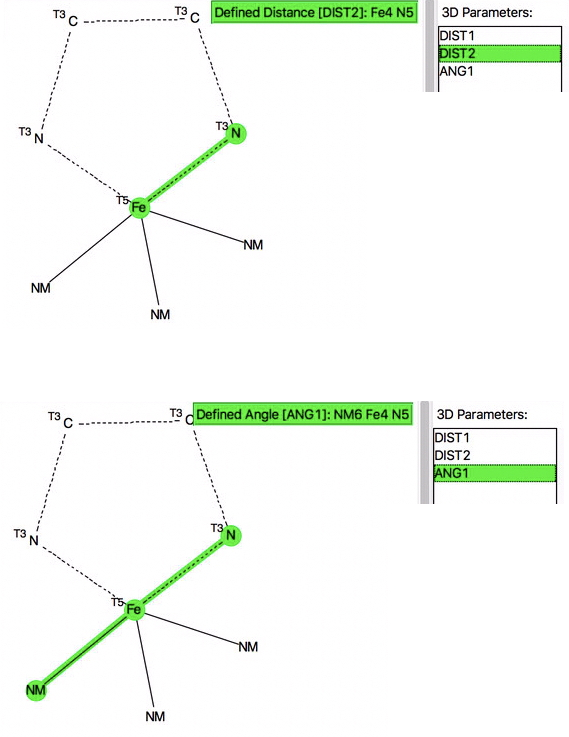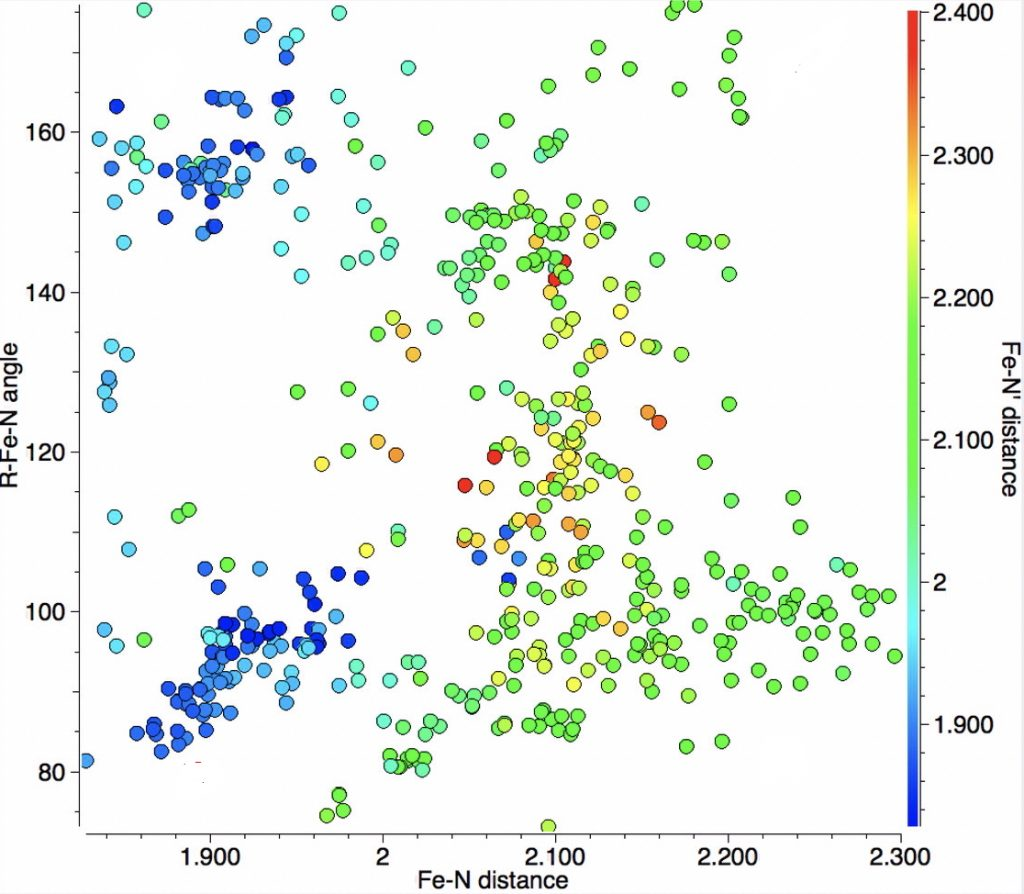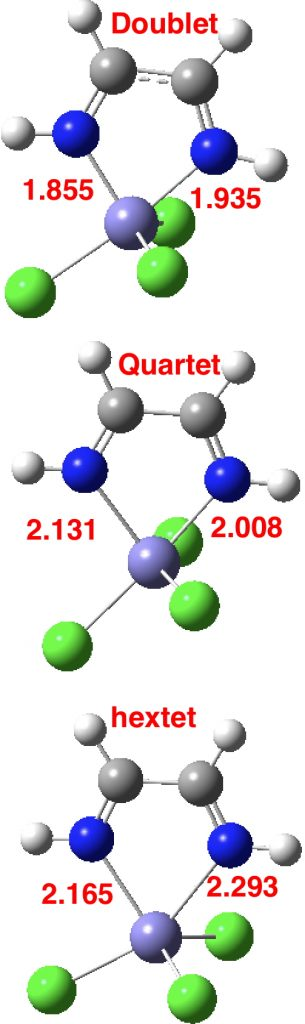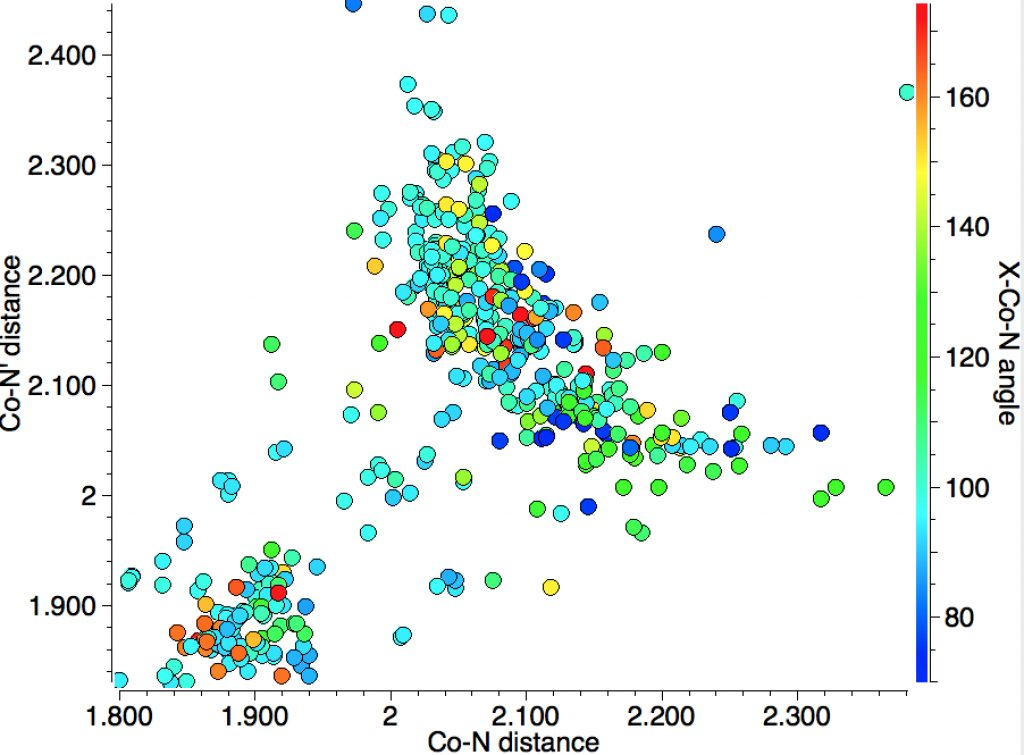## Chemistry rich diagrams: do crystal structures carry spin information? Iron-di-imine complexes.

The iron complex shown below forms the basis for many catalysts. With iron, the catalytic behaviour very much depends on the spin-state of the molecule, which for the below can be either high (hextet) or medium (quartet) spin, with a possibility also of a low spin (doublet) state. Here I explore whether structural information in crystal structures can reflect such spin states.We studied this a few years back and the talk I gave on the topic included some of our first statistical explorations of the CSD (Cambridge structure database). Here I update those searches, using the search query (DOI: 10.14469/hpc/2675) shown below. The di-imine ligand contains only 3-coordinate atoms, whilst the iron is 5-coordinate. The angles subtended at the Fe and group X=NM (any non-metal atom) are as defined below.The resulting scatterplot is shown below and contains a rich variety of phenomena.1. In the bond length region of 1.85-1.95Å one sees three clusters, one arranged on the diagonal indicating both N-Fe lengths are the same and two off the diagonal which indicates one length is ~0.1Å longer than the other.
• To explain this, one needs to know that 5-coordinate Fe has a trigonal bipyramidal shape in which one X=NM group subtends an (anti-periplanar)  angle of ~180° at Fe with one of the ring nitrogens and the other two X=NM groups each subtend an angle of <120° with the other ring nitrogen. The result is that if the group X has the appropriate (electron withdrawing) properties, the two N-Fe bond lengths are no longer equal. If group X is more passive, the two N-Fe bond lengths may remain more equal.2. A second cluster occurs at ~2.00-2.1Å, mostly along the diagonal but with hints of smaller off-diagonal clusters.
3. A third feature occurs at ~2.1-2.3Å, where now the off-diagonal clusters contain more examples than are on the diagonal itself.

Clearly, there is more going on here than can be explained simply by the orientation of X=NM with respect to the Fe-N bond axis. That something is the spin-multiplicity of the molecule. With the Fe complex shown above, this can be one of doublet (one unpaired electron), quartet (three unpaired electrons) or hextet (five unpaired electrons). To gain insight into how this affects the bond lengths, some calculations are needed, using X=Cl, R=H. Here they are done at the TPSSH/Def2-TZVPP level. In fact it is well-known that the energy separations of low/medium/high spin Fe complexes are highly sensitive to the functional, but TPSSH seems to be amongst the best. This shows that the energy ordering of the three states using this particular method is hextet (0.0, DOI: 10.14469/hpc/2676) < quartet (10.5, DOI: 10.14469/hpc/2677) < doublet (13.2 kcal/mol, DOI: 10.14469/hpc/2678), with the bond lengths shown below (for X=Cl).We might make tentative hypotheses based on these values:

1. The off-diagonal bottom left clusters (1 in list above) might arise from doublet states.
2. The off-diagonal top right clusters (3 in list above) might arise from sextet states.
3. The cluster (2 in list above) might be quartet states for which X is not sufficiently electronegative to induce bond length discriminations.
4. It is worth noting that the energy span between the three states for the above molecule is only ~13 kcal/mol, which is small enough to be altered by substituents.

Testing these hypotheses requires knowledge of the spin state of all the entries in any cluster. This information is unfortunately not carried by the CSD, which has relatively little information over and above structural data. Each entry would have to be individually inspected. Indeed the spin state of many of these complexes may not even be known. Nevertheless, it would be great to repeat the graphs shown above as a function of known spin state so that the (again I repeat tentative) hypotheses might be confirmed or refuted.

This article evaluates a whole host of functionals against e.g. the spin-state energy separations of the Fe2+ ion. As it happens, TPSSH was not one that was evaluated, but in fact it gives more or less the best match to experiment. Thus Esinglet-Equintet obs = 85.6 kcal/mol, calc 92.4; Etriplet-Equintet obs 56.1, calc 59.5 kcal/mol. A hypothesis therefore is that the TPSSH functional is a reasonable one to go exploring such high-spin species.

## References

1. M.P. Shaver, L.E.N. Allan, H.S. Rzepa, and V.C. Gibson, "Correlation of Metal Spin State with Catalytic Reactivity: Polymerizations Mediated by α‐Diimine–Iron Complexes", Angewandte Chemie International Edition, vol. 45, pp. 1241-1244, 2006. http://dx.doi.org/10.1002/anie.200502985
2. P. Verma, Z. Varga, J.E.M.N. Klein, C.J. Cramer, L. Que, and D.G. Truhlar, "Assessment of electronic structure methods for the determination of the ground spin states of Fe(ii), Fe(iii) and Fe(iv) complexes", Physical Chemistry Chemical Physics, vol. 19, pp. 13049-13069, 2017. http://dx.doi.org/10.1039/C7CP01263B

### One Response to “Chemistry rich diagrams: do crystal structures carry spin information? Iron-di-imine complexes.”

1.Henry Rzepa says:

Here are the corresponding plots for Mn and Co, adjacent to Fe. Both seem to show distributions associated with three spin states. For Co, the asymmetry in the Co-N bond lengths appears far greater than for Mn, especially for the putative high-spin state.